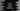# How to import math class in Java example## How to import math class in Java:

Math is an important class in Java that holds a lot of important methods and constants. For example, we can use this class to do logarithm, square root, and trigonometric calculations using its built in methods.

It is a public final class:

``public final class Math``

It belongs to java.lang package.

All methods and variables of this class are static. So, we can easily import these without using any import statements.

In this post, I will show you two different ways to import Math class in a Java program.

### Method 1: Import without using any import statement:

We can use any method or constants defined in the Math class by using the class name. We don’t have to import it because java.lang package is the default package in a Java program.

For example:

``````class Main {
public static void main(String[] args) {
System.out.println(Math.PI);
System.out.println(Math.E);

System.out.println(Math.sin(30));
System.out.println(Math.cos(60));
System.out.println(Math.tan(30));

System.out.println(Math.min(10, 3));
System.out.println(Math.max(10, 20));

System.out.println(Math.sqrt(100));
System.out.println(Math.cbrt(1000));
}
}``````

This class uses different constants and methods of the Math class without using import since all of these methods and constants are static.

If you run this program, it will print the below output:

``````3.141592653589793
2.718281828459045
-0.9880316240928618
-0.9524129804151563
-6.405331196646276
3
20
10.0
10.0``````

### Method 2: Import using static import:

We can also use static import to import the members of the Math class. We can import all methods and constants or we can import only specific members.

If you use static import, we don’t have to use Math class to access.

#### Import specific members:

For example, the below program uses static import to import only PI and E.

``````import static java.lang.Math.PI;
import static java.lang.Math.E;

class Main {
public static void main(String[] args) {
System.out.println(PI);
System.out.println(E);
}
}``````

import static statements are used for static import. If you run this program, it will print the values of PI and E as defined in java.lang.Math class.

``````3.141592653589793
2.718281828459045``````

Note that we don’t have to use the classname if we use static import.

#### Static import all members:

Instead of importing specific members, we can also use * to import everything defined in a class.

For example:

``````import static java.lang.Math.*;

class Main {
public static void main(String[] args) {
System.out.println(PI);
System.out.println(E);
}
}``````

We can use anything defined in the Math class if we use * to import all.

Overloading methods can be used in a similar way. We can use these methods directly and based on the parameters, it will pick the specific method.

``````import static java.lang.Math.*;

class Main {
public static void main(String[] args) {
System.out.println(min(10, 20));
System.out.println(min(10.0, 20.0));
}
}``````

Here, the first method calls:

``public static int min(int a, int b)``

and the second method calls:

``public static long min(long a, long b)``

We don’t have to define specifically, it will automatically decide which method to call based on the parameters.

If you run this program, it will print:

``````10
10.0``````

The first is an integer and the second is a long.